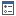Nevron Open Vision Documentation
Properties

In This Topic
In This Topic

The following tables list the members exposed by INLinearGradient.

Public Properties
NameDescriptionGets or sets the End Point X coordinate factor. The coordinate is relative to the gradient rectangle. 0 = left, 1 = right.Gets or sets the End Point Y coordinate factor. The coordinate is relative to the gradient rectangle. 0 = top, 1 = bottom.Gets or sets the Start Point X coordinate factor. The coordinate is relative to the gradient rectangle. 0 = left, 1 = right.Gets or sets the Start Point Y coordinate factor. The coordinate is relative to the gradient rectangle. 0 = top, 1 = bottom.
Top